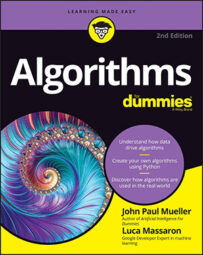##### Algorithms For DummiesGreedy algorithms come in handy for solving a wide array of problems, especially when drafting a global solution is difficult. Sometimes, it's worth giving up complicated plans and simply start looking for low-hanging fruit that resembles the solution you need. In algorithms, you can describe a shortsighted approach like this as greedy.

Looking for easy-to-grasp solutions constitutes the core distinguishing characteristic of greedy algorithms. A greedy algorithm reaches a problem solution using sequential steps where, at each step, it makes a decision based on the best solution at that time, without considering future consequences or implications.

Two elements are essential for distinguishing a greedy algorithm:

• At each turn, you always make the best decision you can at that particular instant.
• You hope that making a series of best decisions results in the best final solution.
Greedy algorithms are simple, intuitive, small, and fast because they usually run in linear time (the running time is proportional to the number of inputs provided). Unfortunately, they don't offer the best solution for all problems, but when they do, they provide the best results quickly. Even when they don't offer the top answers, they can give a nonoptimal solution that may suffice or that you can use as a starting point for further refinement by another algorithmic strategy.

Interestingly, greedy algorithms resemble how humans solve many simple problems without using much brainpower or with limited information. For instance, when working as cashiers and making change, a human naturally uses a greedy approach. You can state the make-change problem as paying a given amount (the change) using the least number of bills and coins among the available denominations.

The following Python example demonstrates the make-change problem is solvable by a greedy approach. It uses the 1, 5, 10, 20, 50, and 100 USD bills, but no coins.

`def change(to_be_changed, denomination):`

```resulting_change = list() ``` `for bill in denomination:`

```while to_be_changed >= bill: ``` ```resulting_change.append(bill) ``` ```to_be_changed = to_be_changed - bill ``` ```return resulting_change, len(resulting_change) ``` ```currency = [100, 50, 20, 10, 5, 1] ``` `amount = 367`

```print ('Change: %s (using %i bills)' ``` `% (change(amount, currency)))`

```Change: [100, 100, 100, 50, 10, 5, 1, 1] (using 8 bills) ``` The algorithm, encapsulated in the `change()` function, scans the denominations available, from the largest to the smallest. It uses the largest available currency to make change until the amount due is less than the denomination. It then moves to the next denomination and performs the same task until it finally reaches the lowest denomination. In this way, `change() `always provides the largest bill possible given an amount to deliver. (This is the greedy principle in action.)

Greedy algorithms are particularly appreciated for scheduling problems, optimal caching, and compression using Huffman coding. They also work fine for some graph problems. For instance, Kruskal's and Prim's algorithms for finding a minimum-cost spanning tree and Dijkstra's shortest-path algorithm are all greedy ones. A greedy approach can also offer a nonoptimal, yet an acceptable first approximation, solution to the traveling salesman problem (TSP) and solve the knapsack problem when quantities aren't discrete.

It shouldn't surprise you that a greedy strategy works so well in the make-change problem. In fact, some problems don't require farsighted strategies: The solution is built using intermediate results (a sequence of decisions), and at every step the right decision is always the best one according to an initially chosen criteria.

Acting greedy is also a very human (and effective) approach to solving economic problems. In the 1987 film Wall Street, Gordon Gecko, the protagonist, declares that "Greed, for lack of a better word, is good" and celebrates greediness as a positive act in economics. Greediness (not in the moral sense, but in the sense of acting to maximize singular objectives, as in a greedy algorithm) is at the core of the neoclassical economy. Economists such as Adam Smith, in the eighteenth century, theorized that the individual's pursuit of self-interest (without a global vision or purpose) benefits society as a whole greatly and renders it prosperous in economy (it's the theory of the invisible hand).

Detailing how a greedy algorithm works (and under what conditions it can work correctly) is straightforward, as explained in the following four steps:

1. You can divide the problem into partial problems. The sum (or other combination) of these partial problems provides the right solution. In this sense, a greedy algorithm isn't much different from a divide-and-conquer algorithm (like Quicksort or Mergesort).
2. The successful execution of the algorithm depends on the successful execution of every partial step. This is the optimal substructure characteristic because an optimal solution is made only of optimal subsolutions.
3. To achieve success at each step, the algorithm considers the input data only at that step. That is, situation status (previous decisions) determines the decision the algorithm makes, but the algorithm doesn't consider consequences. This complete lack of a global strategy is the greedy choice property because being greedy at every phase is enough to offer ultimate success. As an analogy, it's akin to playing the game of chess by not looking ahead more than one move, and yet winning the game.
4. Because the greedy choice property provides hope for success, a greedy algorithm lacks a complex decision rule because it needs, at worst, to consider all the available input elements at each phase. There is no need to compute possible decision implications; consequently, the computational complexity is at worst linear O(n). Greedy algorithms shine because they take the simple route to solving highly complex problems that other algorithms take forever to compute because they look too deep.